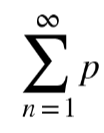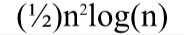# Nth Prime Series Summation

This isn’t an atheist topic. I am posting this here because I believe that to be a theist you have to be somewhat dumb in that you accept the existence of that which has no proof. and to be an atheist you use your intelligence to reject that same premise which defines the theists.

So here is a mathematical concept I worked on and developed. It is the nth prime series summation - ie. 2 + 3 + 5 + 7 + 11 + … + nth prime.

So I’ve worked out a way to approximate the sum given the nth prime. It’s just a conversation piece. Here it is for anyone interested.

When n = 1 and p = 2: what is the numeric value of this piece?@Nyarlathotep

So we begin with the inside of the root. Since n = 1, the value inside the root will be one - because 1^x = 1 for all x.

Now, where you and I got off on the wrong foot was identifying the index for the root. It was mistaken as a multiple and I understand how that could happen, but I assure you I wasn’t trolling you and if anything I approached you first with my formula because of the high regard I hold you in.

That being said. The rest of the calculation is as follows.

For the sake of clarity we can write the remaining calculation as (1)^(1/y) - where “y” is the quite long and complicated index of the root.

Once again, because 1 to any power is 1, the resulting calculation gives us a value of 1 for n=1 and p=2.

I should say that the notation is incorrect. As get off my lawn pointed out to me, the sigma notation implies that I am taking the calculation all the way to infinity. I am not. What I thought it meant when I put infinity above the sigma was for any value of n ranging from 1 to infinity, we can evaluate the approximation given the ensuing formula.

Well for starters, you can’t seem to answer questions about it. For example:

Look, I don’t understand your question. So make it clearer, or don’t bother asking.

Do you want to know the numerical value of the nth prime summation for n = 1, p = 2?

If you don’t, you’re MORE THAN WELCOME to expand so that I understand your question as opposed to you maliciously accusing me of trolling because I don’t know what the fuck you’re asking.

It’s “2”. When n = 1 and p = 2 the series summation is “2”.

Look? Question answered? No? He’s a troll! Or maybe he doesn’t know what you’re getting at and you won’t ELABORATE because your main goal is to trollify me.

Anyway. “2” happy?

The left hand side evaluates to 2 + 2 + 2 + 2 + … = 2 * ∞ = ∞

The right hand side evaluates to 1. Leaving us with:

∞ ≈ 1

eta:
also could you help me with this one:
When n = 2, what is the numerical value of the output of this function:Okay. The notation is not proper. It should be (and I’m not a genius here) the case where sub sigma is (n = 1) and sup. Sigma is “k” where “k = 1 to infinity”.

I THINK you know exactly what I mean by this formula and YES I have to go back and fix the Sigma notation.

But if you’re going to discount the functionality of my approximation because of a notation error on the sigma function - well, that’s in bad faith, my friend.

You’re discounting the whole formula on a technicality.

I did not mean to add 2 an infinite number of times. If you could ACTUALLY help me improve the sigma notation. - well that would be the sign of a gracious player.

If you don’t want to, then ignore the whole thing. I mean after all - it only improves on (1/2 n^2 log n) by a factor of two at least.

The numerical value is .602 and then some EXACTLY as I’ve catalogued in my table of values. You can see for your self.

Its the sum from i = 1 to i =n of Pi| Pi is the first prime when i =1, the 2nd prime when i =2,…,the nth prime when i = n.

1 Like

Well. If I hadn’t thanked you for the input, I will now.

And if I haven’t updated the sigma notation it is purely because I work shitty graveyard shifts and am usually sleeping at times when I could correct the formula.

The sigma notation correction is a quick fix. I fucked up. I get it. So, correct it. Sorry. Can’t. Currently sleeping.

Did Ramanujan give proofs of his result. I’m like a carpenter when it comes to math. I manipulate and bend every last variable until it fits the pattern.

There’s nothing neat and tidy about the result I derived, except that it produces a nice result up to a given value of n.

My lack of python software stops me from calculating the exact value of the series summation beyond what I’ve given in the table of values. I literally don’t have access to any more data.

Thank you. If I ever manage to beat my depression and wake up at a reasonable hour, then I will make the fix.

Yours Sincerely,

Rat spit

You know how I know you aren’t serious? You’ve had like 5+ months to fix it, but you haven’t; but had plenty of time to come here and push a version you knew was wrong.

Now for those people who aren’t math people: I have a question for you guys:

If you were asked to estimate a value, and were given two methods (below) knowing both are essentially guaranteed to be wrong, but method 2 is believed to be slightly less wrong than method 1. Which would you choose:

Method 1:Method 2:

I’m lazy. And not a professional mathematician.

Failure to Launch Fallacy

Then use the simple approximation. The best mathematician is the lazy one.

Your search - “Failure to Launch Fallacy” - did not match any documents.

You sound like a robot

I am destined then (characters)